Download PresentationDisjoint Sets

# Disjoint Sets - PowerPoint PPT Presentation

Disjoint Sets. Jay Chen New York University – Abu Dhabi. 1. Issues:. The equivalence problem The first algorithm Smart union algorithms Union and Find. 2. Equivalence Relations.I am the owner, or an agent authorized to act on behalf of the owner, of the copyrighted work described.
Download Presentation## Disjoint Sets

An Image/Link below is provided (as is) to download presentation

Download Policy: Content on the Website is provided to you AS IS for your information and personal use and may not be sold / licensed / shared on other websites without getting consent from its author.While downloading, if for some reason you are not able to download a presentation, the publisher may have deleted the file from their server.

- - - - - - - - - - - - - - - - - - - - - - - - - - E N D - - - - - - - - - - - - - - - - - - - - - - - - - -
Presentation TranscriptDisjoint Sets

Jay Chen

New York University – Abu Dhabi

1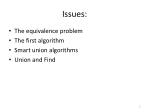Issues:

• The equivalence problem
• The first algorithm
• Smart union algorithms
• Union and Find

2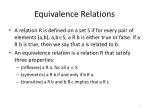Equivalence Relations

• A relation R is defined on a set S if for every pair of elements (a,b), a,bS, a R b is either true or false. If a R b is true, then we say that a is related to b.
• An equivalence relation is a relation R that satisfy three properties:
• (reflexive) a R a, for all a  S.
• (symmetric) a R b if and only if b R a.
• (transitive) a R b and b R c implies that a R c.

3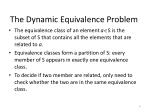The Dynamic Equivalence Problem

• The equivalence class of an element aS is the subset of S that contains all the elements that are related to a.
• Equivalence classes form a partition of S: every member of S appears in exactly one equivalence class.
• To decide if two member are related, only need to check whether the two are in the same equivalence class.

4Disjoint Sets

• Make the input a collection of N sets, each with one element.
• All relations (except reflexive) are false;
• Each set has a different element: SiSj= => it makes the sets disjoint.
• Find operation: returns the name of the set containing a given element.
• Add/Union operation (e.g., add relation a~b)
• Check if a and b are already related: if they are in the same equivalence class.
• If not, apply “union”: merge the two equivalence classes containing a and b into a new equivalence class.

5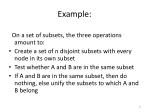Example:

On a set of subsets, the three operations amount to:

• Create a set of n disjoint subsets with every node in its own subset
• Test whether A and B are in the same subset
• If A and B are in the same subset, then do nothing, else unify the subsets to which A and B belong

6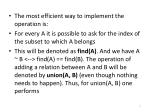The most efficient way to implement the operation is:

• For every A it is possible to ask for the index of the subset to which A belongs
• This will be denoted as find(A). And we have A ~ B <--> find(A) == find(B). The operation of adding a relation between A and B will be denoted by union(A, B) (even though nothing needs to happen). Thus, for union(A, B) one performs

7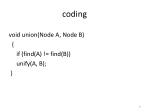coding

void union(Node A, Node B)

{

if (find(A) != find(B))

unify(A, B);

}

8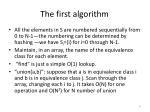The first algorithm

• All the elements in S are numbered sequentially from 0 to N-1—the numbering can be determined by hashing —we have Si={i} for i=0 through N-1.
• Maintain, in an array, the name of the equivalence class for each element.
• “find” is just a simple O(1) lookup.
• “union(a,b)”: suppose that a is in equivalence class i and b is in equivalence class j. Scan through the array, changing each i to j. It takes O(N) for one operation and O(N2) for N number of union

9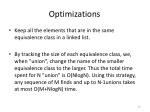Optimizations

• Keep all the elements that are in the same equivalence class in a linked list.
• By tracking the size of each equivalence class, we, when “union”, change the name of the smaller equivalence class to the larger. Thus the total time spent for N “union” is O(NlogN). Using this strategy, any sequence of M finds and up to N-1unions takes at most O(M+NlogN) time.

10The O(M+N) algorithm

Class DisjSets

{

pubic:

explicit DisjSets(int numElements);

int find(int x) const;

int find(int x);

void unionSets(int root1,int root2);

private:

vector<int> s;

}

14The O(M+N) algorithm

DisjSets::DisjSets(int numElements):s(numElements)

{

for(int j=0; j<s.size(); j++)

s[j]=-1;

}

Void DisjSets::unionSets(root1, root2)

{

s[root2]=root1;

}

15The O(M+N) algorithm

DisjSets:: find(int x) const

{

if(s[x]<0)

return x;

else

return find(s[x]);

}

16Smart Union Algorithms

• Union-by-size: make the smaller tree a subtree of the larger.
• If union-by-size, the depth of any node is never more than logN: a find operation is O(logN), and O(MlogN) for a sequence of M. The worse-case trees are binomial trees.

17Path Compression for faster find

• Path compression for find(x): every node on the path from x to the root has its parent changed to the root: make the tree shallow.

20Smart Union Algorithms

/* Union-by-height: make the shallow tree a subtree of the deeper. */

void DisjSets::unionSets(root1, root2)

{

if(s[root2]<s[root1]) //root2 is deeper

s[root1]=root2;

else { //update height if same

if(s[root1]==s[root2])

s[root1]--;

s[root2]=root1;

}

}

21DisjSets:: find(int x)

{

if(s[x]<0)

return x;

else

return s[x]=find(s[x]);

}

22Union and Find

The Union-Find problem starts with n elements (numbered 1 to n), each one representing a singleton set. We allow two operations to be performed:

23Find (i) returns the ID number of the set that i

currently is in. We assume the ID number of

each set is the number of one of the elements

in it.

• Union (i; j) combines the elements in the sets

with ID numbers i and j into a single set.

The ID number of the new set will be either

i or j . This is a destructive operation in the

sense that the original sets i and j are lost

when they are combined to form the new set.

24We can implement Union-Find by maintaining

an array A[1 : : : n] of integers. If i is the ID

number of a set, then A[i] will be the negative

of the number of elements in this set. Otherwise, A[i] will the number of another element in this set.

• We begin with each A[i] equal to -1The

Union operation is easily implemented as follows:

25void Union (int i, int j)

{ if (A [i] < A [j])

{ A [i] += A [j];

A [j] = i; }

else

{ A [j] += A [i];

A [i] = j; }

return;

}

This simply makes the \leader" of the smaller

set point to the leader of the larger set, and

adjusts the size of the leader. The time complexity

is O(1)

26To implement Find (i) we just follow the pointers

int Find (int i)

{

while (A [i] > 0)

i = A [i];

return i;

}

This yields O(lg n) time. We can do better, though, with path compression. After we find the leader, we make all the nodes we've passed through point directly to it:

27int Find (int i)

{

int j = i, k;

if (A [i] < 0)

return i;

while (A [j] > 0)

j = A [j];

while (A [i] != j)

{

k = A [i];

A [i] = j;

i = k;

}

return j;

}

28Using Union by size (rank) and Find with path compression, we get the following result:

Starting with n singleton sets, any sequence of M Union and/or Find operations takes O(M logN) time.

29